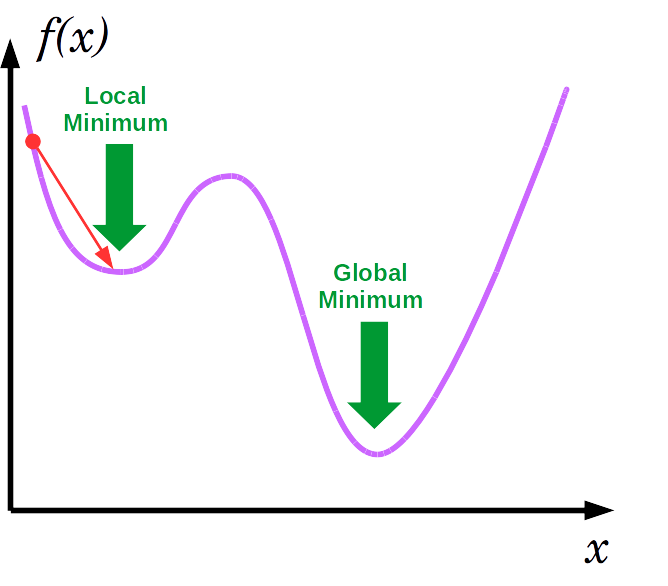×

Search anything:

# Simulated Annealing

#### data science Machine Learning (ML)Get this book -> Problems on Array: For Interviews and Competitive Programming

In this article, we will understand what simulated annealing is and get to know its uses in Probability and Data Science.

• What is simulated annealing?
• Algorithm

# What is simulated annealing?In a situation like shown above, the gradient descent gets stuck at the local minima if it started at the indicated point. It wouldn't further be able to reach the global minima. In cases like these, simulated annealing proves useful.

Simulated annealing is an algorithm based on the physical annealing process used in metallurgy. During physical annealing, the metal is heated up until it reaches its annealing temperature and then is gradually cooled down to change it into the desired shape. It is based on the principle that the molecular structure of the metal is weak when it is hot and can be changed easily. Where as when it cools down, it becomes hard and thus changing the shape of the metal becomes difficult.

Simulated annealing has a probabilistic way of moving around in a search space and is used for optimizing model parameters. It mimics physical annealing as a temperature parameter is used here too.

If the temperature is higher, the more likely the algorithm will accept a worse solution. This expands the search space unlike gradient descent and allows it to travel down a trivial path. This promotes exploration.

If the temperature is lower, the less likely it will accept a worse solution. This tells the algorithm that once it is in the right part of the search space, it does not need to search any other parts and instead must focus on finding the global maximum by converging. This promotes exploitation.

The main difference between a greedy search and simulated annealing is that the greedy search always go for the best option where as in simulated annealing, it has a probability (using Boltzmann distribution) to accept a worse solution.

# Algorithm

For a function h(•) we are trying to maximize, the steps for simulated annealing algorithm is as follows:

1. Start by generating an initial solution x.
2. Set the initial temperature t=t0 where t0 > 0.
3. For the n number of iterations i=1,2,...,n , loop through the following steps until the termination condition is reached:
• Sample out θ ~ g(θ) where g is a symmetric distribution.

• The new candidate solution becomes x'=x ± θ.

• We find the difference in cost between our old and new solution (Δh=h(x')-h(x)) and calculate the probability p using the difference and the current temperature(ti). This is the probability that we should accept/ reject the candidate solution.

p= exp(Δh/ti)

• If Δh is greater than zero, it means that our new solution is better and we accept it. If it is less than zero, then we generate a random number u ~ U(0,1). We accept the new solution x' if u ≤ p.

• We then reduce the temperature t using a temperature reduction function α. Temperature reduction functions like t = t - α or t = t * α may be used here.

The termination conditions here may be achieving a particular temperature or a performance threshold.

Note that if the temperature is high, say maybe a 100, then the probability that we are going to accept the candidate solution comes out to be high, when we substitute it in the formula. As the temperature becomes closer to 0, the algorithm functions like the greedy hill climbing algorithm.

## Advantages of Simulated Annealing

• Simulated annealing is easy to code and use.
• It does not rely on restrictive properties of the model and hence is versatile.
• It can deal with noisy data and highly non-linear models.
• Provides optimal solution for many problems and is robust.

## Disadvantages of Simulated Annealing

• A lot of parameters have to be tuned as it is metaheuristic.
• The precision of the numbers used in its implementation has a significant effect on the quality of results.
• There is a tradeoff between the quality of result and the time taken for the algorithm to run.

With this article at OpenGenus, you must have the complete idea of Simulated Annealing.#### Sanjana Babu

Sanjana Babu is a Student at SRM Valliammai Engineering College and is an Intern at OpenGenus. She is a Philomath.